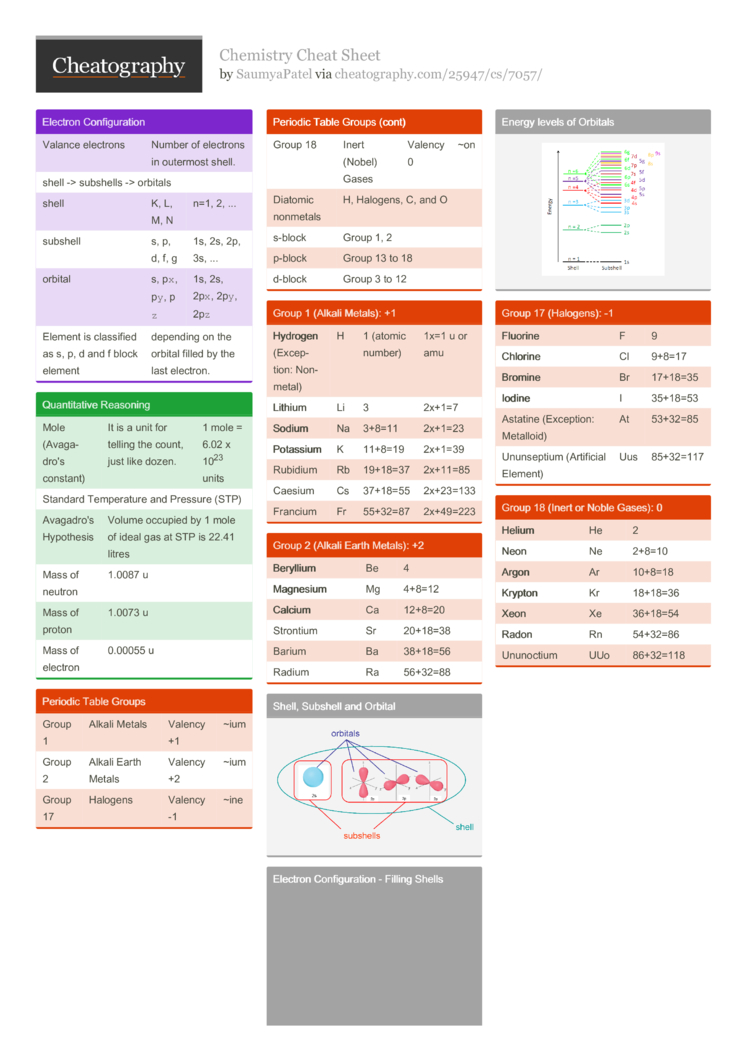# Chemistry Cheat Sheet by SaumyaPatel

### Electron Config­uration

 Valance electrons Number of electrons in outermost shell. shell -> subshells -> orbitals shell K, L, M, N n=1, 2, ... subshell s, p, d, f, g 1s, 2s, 2p, 3s, ... orbital s, p`x`, p`y`, p`z` 1s, 2s, 2p`x`, 2p`y`, 2p`z` Element is classified as s, p, d and f block element depending on the orbital filled by the last electron.

### Quanti­tative Reasoning

 Mole (Avaga­dro's constant) It is a unit for telling the count, just like dozen. 1 mole = 6.02 x 1023 units Standard Temper­ature and Pressure (STP) Avagadro's Hypothesis Volume occupied by 1 mole of ideal gas at STP is 22.41 litres Mass of neutron 1.0087 u Mass of proton 1.0073 u Mass of electron 0.00055 u

### Periodic Table Groups

 Group 1 Alkali Metals Valency +1 ~ium Group 2 Alkali Earth Metals Valency +2 ~ium Group 17 Halogens Valency -1 ~ine Group 18 Inert (Nobel) Gases Valency 0 ~on Diatomic nonmetals H, Halogens, C, and O s-block Group 1, 2 p-block Group 13 to 18 d-block Group 3 to 12

### Group 1 (Alkali Metals): +1

 Hydr­ogen (Excep­tion: Non-metal) H 1 (atomic number) 1x=1 u or amu Lith­ium Li 3 2x+1=7 Sodium Na 3+8=11 2x+1=23 Pota­ssium K 11+8=19 2x+1=39 Rubidium Rb 19+18=37 2x+11=85 Caesium Cs 37+18=55 2x+23=133 Francium Fr 55+32=87 2x+49=223

### Group 2 (Alkali Earth Metals): +2

 Bery­llium Be 4 Magn­esium Mg 4+8=12 Calc­ium Ca 12+8=20 Strontium Sr 20+18=38 Barium Ba 38+18=56 Radium Ra 56+32=88

### Shell, Subshell and Orbital### Electron Config­uration - Filling Shells### Energy levels of Orbitals### Group 17 (Halog­ens): -1

 Fluo­rine F 9 Chlo­rine Cl 9+8=17 Brom­ine Br 17+18=35 Iodine I 35+18=53 Astatine (Excep­tion: Metalloid) At 53+32=85 Ununse­ptium (Artif­icial Element) Uus 85+32=117

### Group 18 (Inert or Noble Gases): 0

 Helium He 2 Neon Ne 2+8=10 Argon Ar 10+8=18 Kryp­ton Kr 18+18=36 Xeon Xe 36+18=54 Radon Rn 54+32=86 Ununoctium UUo 86+32=1181 Page
//media.cheatography.com/storage/thumb/saumyapatel_chemistry.750.jpg

PDF (recommended)